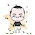## Sunday, July 14, 2013

### Vintage Years in Econometrics - The 1950's

Following on from my earlier posts about vintage years for econometrics in the 1930's and 1940's, here's my run-down on the 1950's.

As before, let me note that "in econometrics, what constitutes quality and importance is partly a matter of taste - just like wine! So, not all of you will agree with the choices I've made in the following compilation."

1950:
• Anderson, T. W. and H. Rubin, The asymptotic properties of the estimators of the parameters of a single equation in a complete system of stochastic equations. Annals of Mathematical Statistics, 21, 570-582.6
• Durbin, J. and G. S. Watson, Testing for serial correlation in least squares regression - I. Biometrika, 37, 409-428.
• Klein, L. R., Economic Fluctuations in the United States, 1921 to 1941. Wiley, New York.1
• Koopmans, T. C. (ed.), Statistical Inference in Dynamic Economic Models. Wiley, New York.2
• Identifiability of a linear relation between variables which are subject to error. Econometrica18, 375–389.
• Waugh, F. V., Inversion of the Leontief matrix by power series. Econometrica, 18, 142-154.
1951:
• Durbin, J. and G. S. Watson, Testing for serial correlation in least squares regression - II. Biometrika, 38, 159-178.
• Wald, A., On some systems of equations of mathematical economics. Econometrica, 19, 368-403.3
1952:
• Brown, T. M., Habit persistence and lags in consumer behaviour. Econometrica, 20, 355–71.
• Klein, L. R., A Textbook of Econometrics, Prentice-Hall, Englewood Cliffs, NJ.
• Tintner, G., Econometrics. Wiley, New York.4
1953:
• Hood, W. C. and T. C. Koopmans (eds.), Studies in Econometric Methods. Wiley, New York.5
• Theil, H., Repeated least-squares applied to complete equation systems. Centraal Planbureau Memorandum.
• Theil, H., Estimation and simultaneous correlation in complete equation systems. Centraal Planbureau Memorandum. (Reprinted In: Raj, B., and J. Koerts (eds.), 1992, Henri Theil’s Contributions to Economics and Econometrics, Vol. 1, Kluwer, Dordrecht.)
1954:
• Koyck, L., Distributed Lags and Investment Analysis. North-Holland, Amsterdam.
• The Measurement of Consumers' Expenditure and Behaviour in the United Kingdom, 1920-1938, vol. 1.7
• Stone, J. R. N., Linear expenditure systems and demand analysis: An application to the pattern of British demand. Economic Journal, 64, 511–527.
• Theil, H., Estimation of parameters of econometric models. Bulletin of International Statistics Institute, 34, 122–128.
1955:
1956:
• Theil, H. and J. van Ijzeren, On the efficiency of Wald's method of fitting straight lines. Review of the International Statistical Institute, 24, 17-26.
1957:
• Basmann,R. L., A generalized classical method of linear estimation of coefficients in a structural equation. Econometrica, 25, 77–83.6
• Friedman, M.A Theory of the Consumption Function.Princeton, Princeton University Press.
• Theil, H., Specification errors and the estimation of economic relationships. Review of the International Statistical Institute, 25, 41-51.
1958:
• Hooper, J. W. and H. Theil, The extension of Wald's method of fitting straight lines to multiple regression, Review of the International Statistical Institute, 26, 37-47.
• Nerlove, M. , Distributed Lags and Demand Analysis for Agricultural and Other Commodities. USDA, Agriculture Handbook No. 141, Washington, DC.
• Nerlove, M., Adaptive expectations and the cobweb phenomena. Quarterly Journal of Economics, 72, 227–240.
• Sargan, J. D., The estimation of economic relationships using instrumental variables. Econometrica, 26, 393–415.6,8
• Tobin, J., Estimation of relationships for limited dependent variables. Econometrica, 26, 24-36.
1959:
• Basmann, R. L., The computation of generalized classical estimates of coefficients in a structural equation. Econometrica, 27, 72-81.
• Hooper, J. W., Simultaneous equations and canonical correlation theory. Econometrica, 27, 245-256.
• Kuh, E., The validity of cross-sectionally estimated behaviour equations in time series applications. Econometrica, 27, 197–214.
• Nagar, A. L., The bias and moment matrix of the general k-class estimators of the parameters in simultaneous equations. Econometrica, 27, 575-595.
• Sargan, J. D., The estimation of relationships with autocorrelated residuals by the use of instrumental variables. Journal of the Royal Statistical Society, B, 21, 91-105.
• Theil, H., The aggregation implications of identfiable structural macro-relations, Econometrica, 27, 14-29.
• Tinbergen, J., Econometrics. George Allen and Unwin, London.

Best vintages of the 1950's - 1950 and 1953!

Tasting Notes:
1. The Cowles Commission monograph that gave us Klein's Model I.
2. This Cowles Commission book contained no less than 18 extremely influential papers in the areas of identification theory, simultaneous equations models and time-series analysis. The chapter by Koopmans, Rubin and Leipnik introduces the term "identification" for the first time, and presents the rank and order conditions for identifiability of a structural equation.
3. Abraham Wald died in 1950. This issue of Econometrica carries his obituary.
4. The first econometrics textbook?
5. Another Cowles Commission book with a star-studded list of contributors. Includes the formal introduction of the FIML estimator by Chernoff and Divinsky.
6. Theil, Basmann, and Sargan are usually credited (separately) with the development of the 2SLS estimator. However, Anderson (2005) has pointed out that the properties of the 2SLS estimator were actually dealt with by Anderson and Rubin (1950).
7. On p. 302 we find the first use of the term "multicollinearity" in its "modern" sense.
8. A truly path-breaking paper that was well ahead of its time. It anticipated much of modern time-series econometrics.

1.Very interesting to see the original works upon which so much of "modern" econometrics is based. This blog is a true public service. Thanks.

1.Thanks. It's fun putting it together.

2.Not econometrics per se, but shapes so much of modern - gulp! - microeconometrics on program evaluation. Roy, A. 1951. Some thoughts on the distribution of earnings. Oxford Economic Papers3, 135–46.

1.